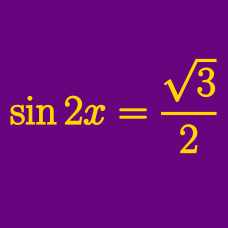Geometry

# Trigonometric Equations - R method

In the interval $[0, 20 \pi ]$, how many solutions are there to

$\sin \theta + \cos \theta = 1?$

What is the maximum value of the function $f(x)=2\sin \left(x+\frac{\pi}{3}\right)+\sqrt{3}\cos x+2 ?$

The sum of all the $\theta$'s in the interval $12\pi < \theta < 14\pi$ such that the quadratic equation for $x$ $x^2-2(\sin \theta+\cos \theta)x+\cos 2\theta=0$ has a repeated root is $\frac{a}{2}\pi$. What is the value of $a?$

If provided that $\sqrt{3}\cos \theta+\sin \theta=\frac{\sqrt{3}}{2}$ and $\displaystyle \frac{\pi}{2} < \theta < \pi,$ what is the value of $\sqrt{3}\sin \theta-\cos \theta ?$

What are the maximum and minimum values of the function $y=\sqrt{2}\sin x-\cos x ?$

×